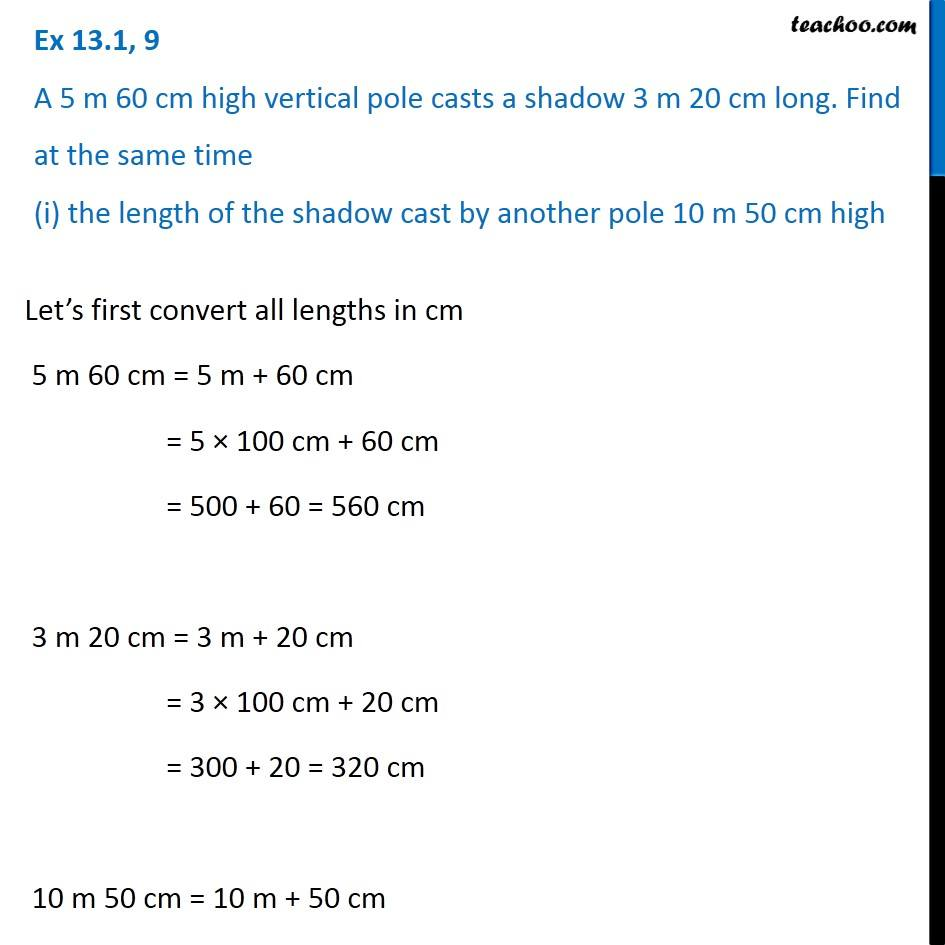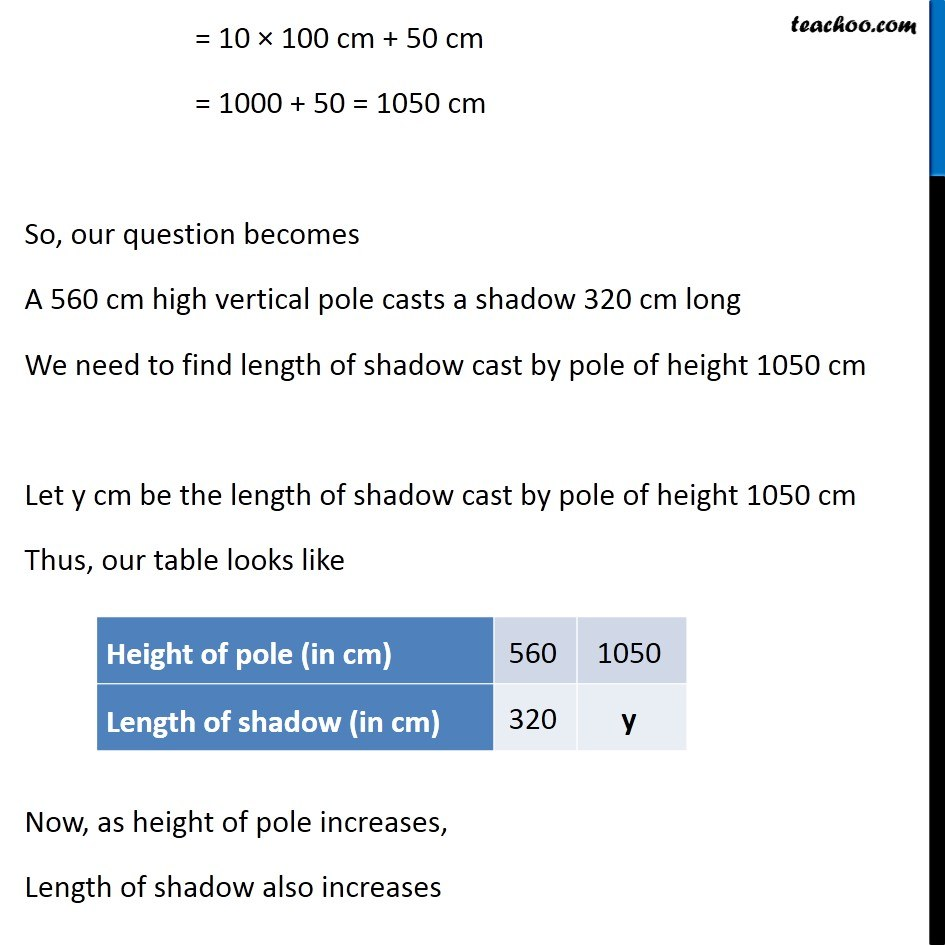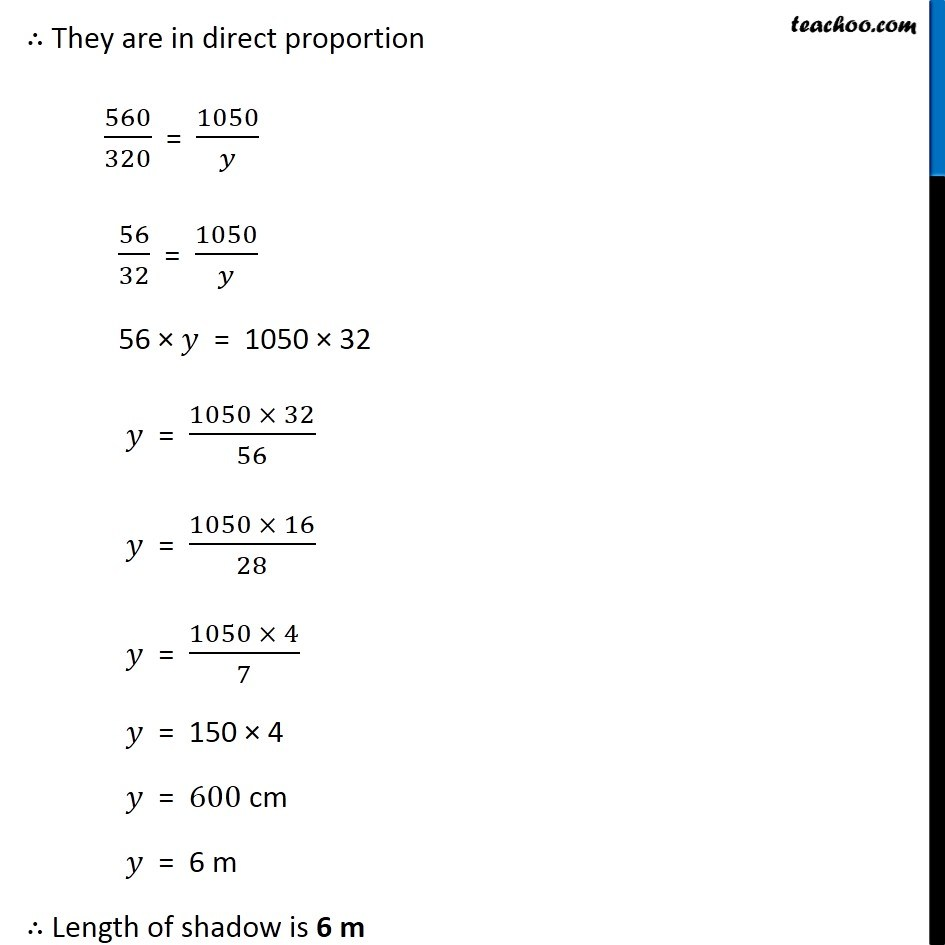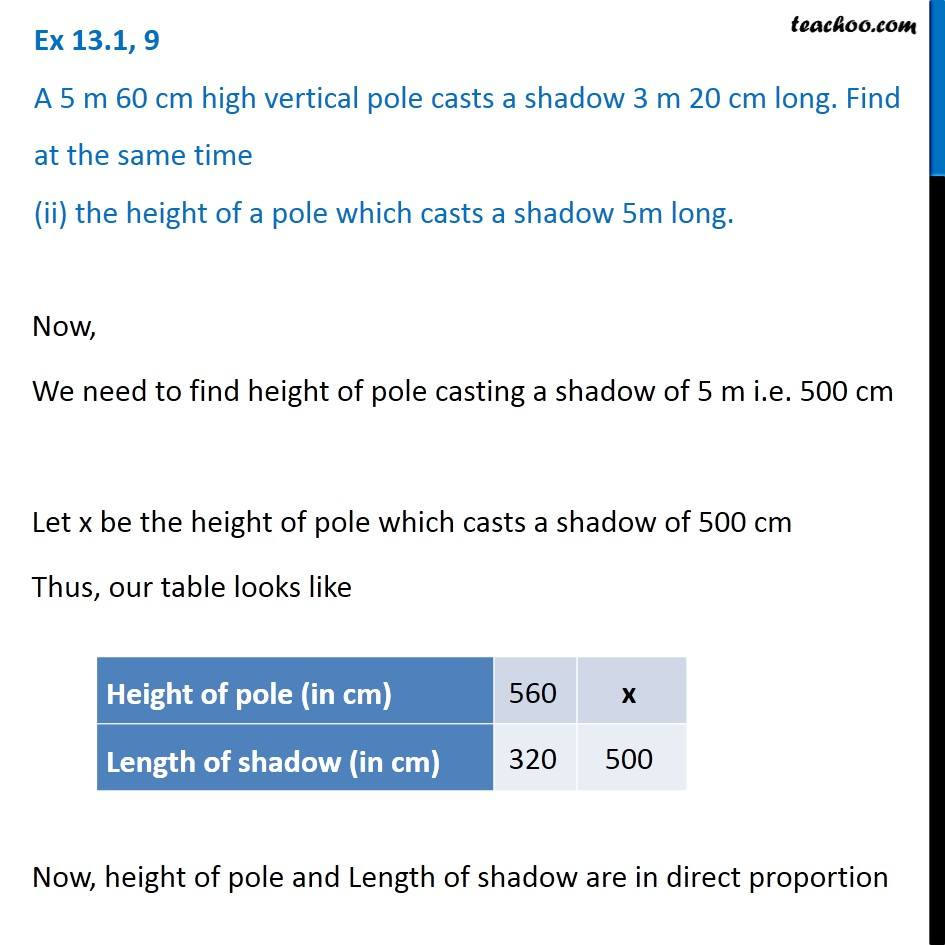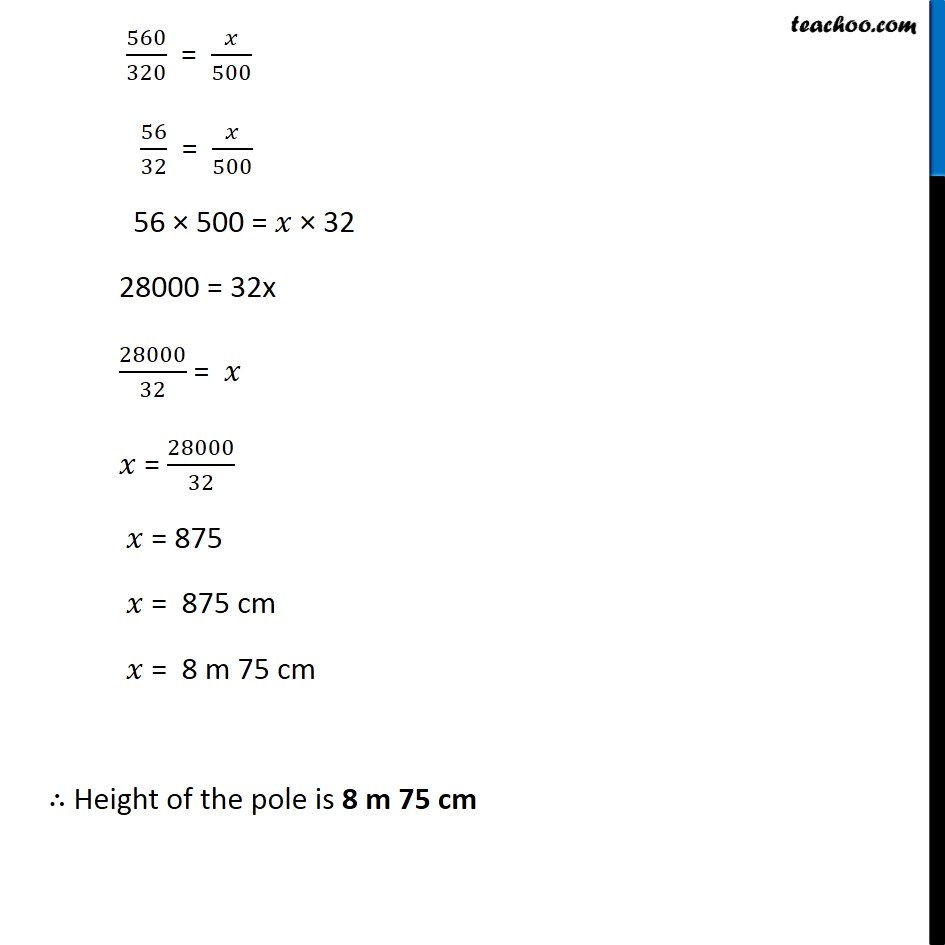1. Chapter 13 Class 8 Direct and Inverse Proportions
2. Serial order wise
3. Ex 13.1

Transcript

Ex 13.1, 9 A 5 m 60 cm high vertical pole casts a shadow 3 m 20 cm long. Find at the same time (i) the length of the shadow cast by another pole 10 m 50 cm high Let’s first convert all lengths in cm 5 m 60 cm = 5 m + 60 cm = 5 × 100 cm + 60 cm = 500 + 60 = 560 cm 3 m 20 cm = 3 m + 20 cm = 3 × 100 cm + 20 cm = 300 + 20 = 320 cm 10 m 50 cm = 10 m + 50 cm = 10 × 100 cm + 50 cm = 1000 + 50 = 1050 cm So, our question becomes A 560 cm high vertical pole casts a shadow 320 cm long We need to find length of shadow cast by pole of height 1050 cm Let y cm be the length of shadow cast by pole of height 1050 cm Thus, our table looks like Now, as height of pole increases, Length of shadow also increases ∴ They are in direct proportion 560/320 = 1050/𝑦 56/32 = 1050/𝑦 56 × 𝑦 = 1050 × 32 𝑦 = (1050 × 32)/56 𝑦 = (1050 × 16)/28 𝑦 = (1050 × 4)/7 𝑦 = 150 × 4 𝑦 = 600 cm 𝑦 = 6 m ∴ Length of shadow is 6 m Ex 13.1, 9 A 5 m 60 cm high vertical pole casts a shadow 3 m 20 cm long. Find at the same time (ii) the height of a pole which casts a shadow 5m long. Now, We need to find height of pole casting a shadow of 5 m i.e. 500 cm Let x be the height of pole which casts a shadow of 500 cm Thus, our table looks like Now, height of pole and Length of shadow are in direct proportion 560/320 = 𝑥/500 56/32 = 𝑥/500 56 × 500 = 𝑥 × 32 28000 = 32x 28000/32 = 𝑥 𝑥 = 28000/32 𝑥 = 875 𝑥 = 875 cm 𝑥 = 8 m 75 cm ∴ Height of the pole is 8 m 75 cm